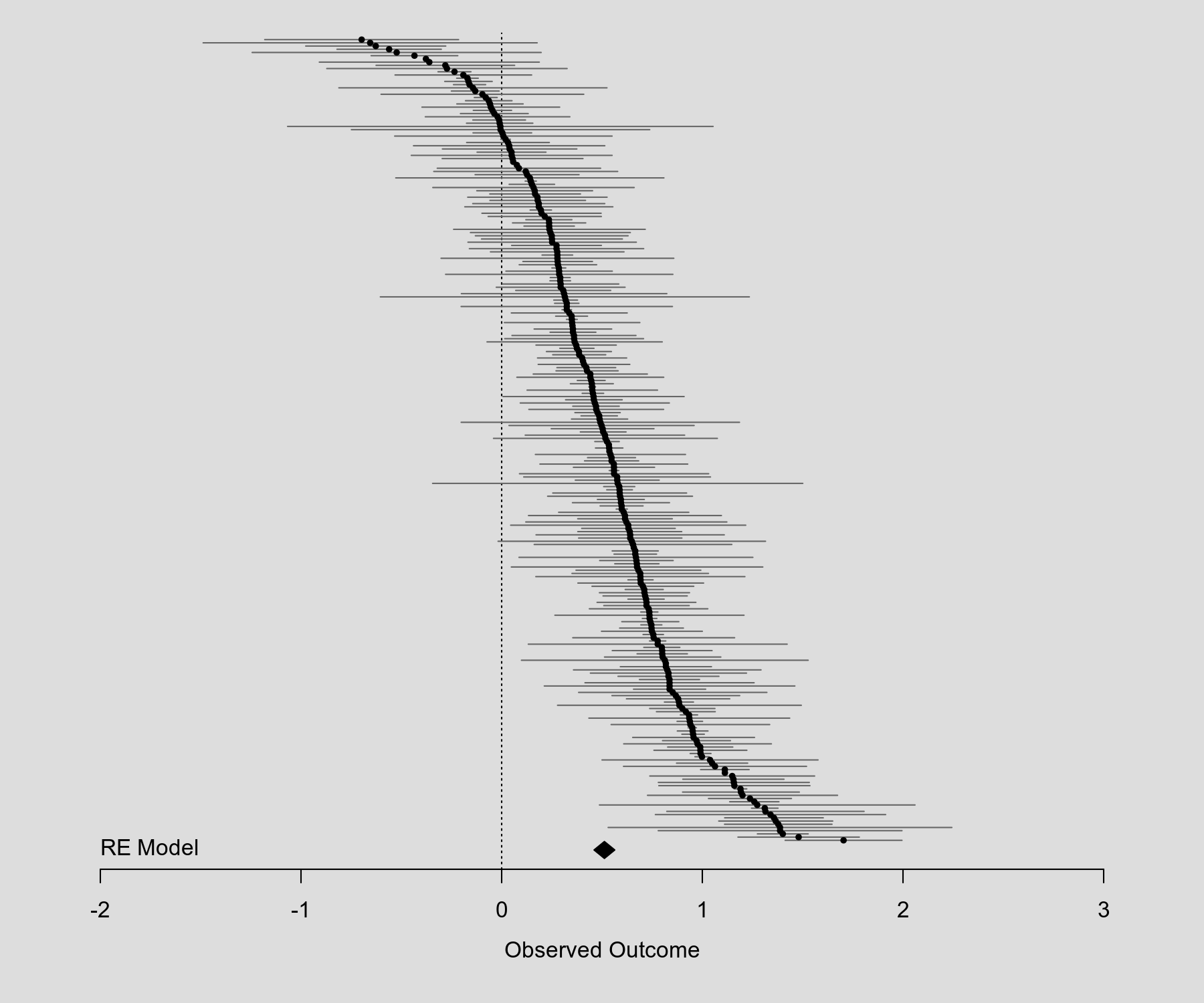#The metafor Package

A Meta-Analysis Package for R

### Sidebar

plots:caterpillar_plot

This is an old revision of the document!

## Caterpillar Plot

### Description

A caterpillar plot is in essence nothing different than what is often called a forest plot in the meta-analytic literature, except that the estimates are ordered by their magnitude. Also, the term tends to be used under circumstances where the number of estimates is rather large, so focus is on showing the general pattern of the results and not making individual estimates fully discernible. The code below shows how such a plot can be created with the forest() function. In case this is needed, one can also add a summary polygon to the bottom of the plot.

### Plot### Code

library(metafor)

### simulate some data
set.seed(5132)
k <- 250
vi <- rchisq(k, df=1) * .03
yi <- rnorm(k, rnorm(k, 0.5, 0.4), sqrt(vi))

### fit RE model
res <- rma(yi, vi)

### create plot
forest(yi, vi,
xlim=c(-2.5,3.5),        ### adjust horizontal plot region limits
subset=order(yi),        ### order by size of yi
slab=NA, annotate=FALSE, ### remove study labels and annotations
efac=0,                  ### remove vertical bars at end of CIs
pch=19,                  ### changing point symbol to filled circle
col="gray40",            ### change color of points/CIs
psize=2,                 ### increase point size
cex.lab=1, cex.axis=1,   ### increase size of x-axis title/labels
lty=c("solid","blank"))  ### remove horizontal line at top of plot

### draw points one more time to make them easier to see
points(sort(yi), k:1, pch=19, cex=0.5)

### add summary polygon at bottom and text
text(-2, -2, "RE Model", pos=4, offset=0, cex=1)